you are here:/ News & Insights / Engineering Advantage Blog / Random Data Analysis Part 2: Intro to the Fast Fourier Transform

# Random Data Analysis Part 2: Intro to the Fast Fourier TransformSeptember 4, 2015 By: Jonathan Dudley

Last time, I discussed the concept of a stochastic process and random data analysis. Specifically, the focus was on computing temporal statistics such as the mean and standard deviation. In this post, I would like to introduce the Fast Fourier Transform (FFT) and its implementation and use in data post-processing of a CFD analysis.

The French mathematician J.B.J. Fourier discovered that periodic waveforms can be modeled as the sum of sine and cosine waves called a Fourier series. The mathematical expression is given in Equation 1 for a generic signal with a range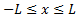. Application of Equation 1 for a square wave with increasing values of “n” yields Figure 1, where it is apparent that as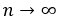the square wave is more accurately reproduced.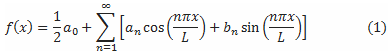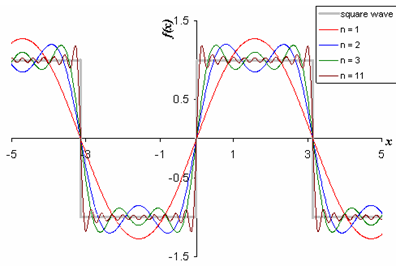The Fourier transform is used to represent a periodic function by a discrete sum of complex exponentials. Instead of Equation 1, we could equally write the summation in complex form: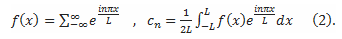If we define a new variable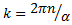we can define the Fourier integral as (after some math)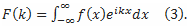If we want a result for F(k), which is called the Fourier transform of f(x), we simply apply equation (2) in the limit as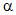approaches infinity with the Fourier series coefficients: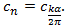The Fourier transform is closely related to a Fourier series and is used to represent a general function by a continuous superposition (or integral) of complex exponentials. The Fourier transform decomposes an arbitrary waveform into its sine components thus revealing it’s frequency content that might else be difficult to detect. A Discrete-Time Fourier transform (DTFT) is a form of the Fourier transform that is applicable to uniformly sampled continuous functions. The Fast Fourier Transform (FFT) takes advantages of powers of two and is a numerically efficient implementation of computing DTFT’s. Simply stated, the FFT is an efficient numerical algorithm that allows for the transformation of a time dependent signal into the frequency domain (or vice-versa).

For illustration purposes, let’s consider a random fluctuating pressure signal with the mean removed as shown in Figure 2 that was used in the previous blog. The data is acquired at a single point in space and sampled at uniform frequency of 1000 [kHz]. It is difficult to extract meaningful information from this signal outside of typical temporal statics like mean and variance that was illustrated in the previous post.

Figure 2: Acquired random pressure signal

## cfd data 2.pngIn order to extract more meaningful information, we pass this series of data through an FFT algorithm. The resulting transform results in what is called a Power-Spectral Density (PSD) plot and is illustrated in Figure 3. The y-axis has been partially non-dimensionalized using the free stream dynamic pressure. Bear in in mind that during the FFT, the resulting units will be based on the signal passed. In this case, since pressure was used, the resulting units are [Pa^2/Hz] and results in a “power spectral density”. The plot  describes the distribution of the mean square power over a frequency range related to the Nyquist frequency. Examining this figure, we can now clearly see at least 5 discrete peaks occur as tabulated in Table 1.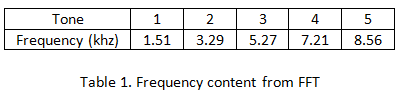Figure 3. FFT of the pressure signal

## cfd data 3.pngFurther, it is clear that the third tone is dominant and exhibits over 3 times the peak power compared to the other tones. The total power could be determined by obtaining the area under the curve with specified frequency intervals if desired, such as with Octave analysis.

Hopefully, this blog sheds some light on how you can make use of an FFT analysis to extract additional information while post-processing uniformly discretely sampled time dependent data series. In my next post, I will breakdown the process for performing the FFT for a given set of data. I will discuss the effects of the input parameters on the outcome of the FFT required to perform the analysis. If you have further experiences or questions please feel free to add a comment.